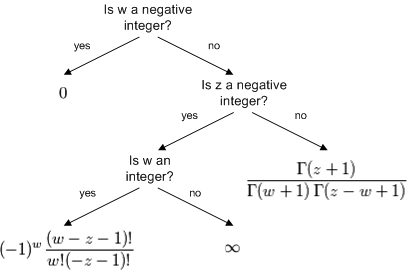# How to calculate binomial coefficients

This article explains how to calculate binomial coefficients for general arguments. See the article binomial coefficients for definitions.

The flow chart below summarizes how to express the binomial coefficient (z, w) in terms of more familiar functions.Note that the expressions at the terminal nodes of the decision tree are only involve taking factorials of positive integers or evaluating the gamma function where it is non-singular.

The Mathematica function `Binomial[z, w]` implements binomial coefficients as explained here. The R function `choose(z, w)` requires z to be real and w to be an integer.

The fractions should be evaluated by subtracting logarithms rather than computing the numerators and denominators directly. Otherwise, the numerators or denominators will overflow for large arguments. See How to calculate binomial probabilities for details.

* * *